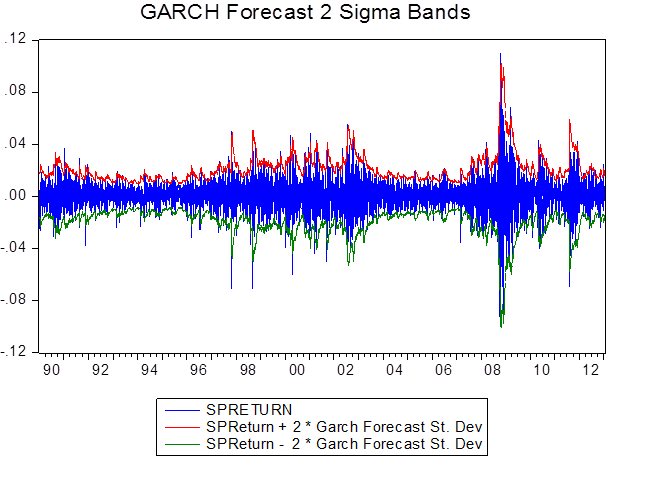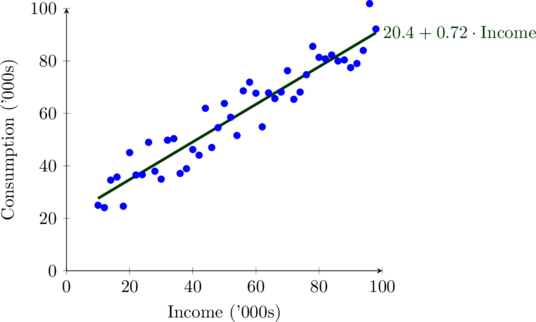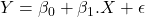# Econometrics Tutor Cardiff University – Top Microeconomics, Macroeconomics Tutors

### Handpicked teachers for Economics from University of Cardiff. Private lessons in Microeconomics, Macroeconomics, Econometrics.Work with our Econometrics Tutor Cardiff University for all economics and finance courses.

Economic theory posits relationships between variables
• Economic theory is usually informative about sign of the relationships…but uninformative about the magnitude of the relationships
• Regression analysis can be used to:
• test the theory, i.e., establish whether there is a relationship and, if there is, whether it is positive or negative; and estimate the magnitude of the relationship
• Regression analysis is a technique for estimating econometric models

The parameters/coefficients in an econometric model capture the relationships between the variables in the model

#### Simple Linear Regressionrepresents the factors other than x that affect Y,
• there may be many such factors
• some of these factors may be unobservable (not possible to measure)
• also accounts for (random) measurement error in variables

u accounts for the factors other than x that affect y , i.e., factors that we have not explicitly accounted for in the model
• How can we obtain an estimate of B the ceteris paribus effect of X on Y, when we are simply ignoring these other factors, by relegating them to u?
• We are not ignoring them: we are going to make several important assumptions about them and it is only if these assumptions are valid that we can interpret our estimate of B as the ceteris paribus effect of  X on Y

### Properties of OLS Estimator

The OLS estimator “finds” the values for B0 and B1 that minimise the sum of the squared vertical distances between each observation (point) and the line

The property of unbiasedness is about the average values of b1 and b2 if many samples of the same size are drawn from the same population.

Here, based on 10 households: b1 = 78:74 and b2 = 9:68. These averages would approach the true parameter values 1 and 2 if we took averages of estimates from many samples

Unbiasedness does not say that an estimate from any one sample is close to the true parameter value, but that the estimator is unbiased. Under the assumptions SR1-SR5 of the linear regression model, the estimators b1 and b2 have the smallest variance of all linear and unbiased estimators of 1 and 2. They are the Best Linear Unbiased Estimators (BLUE) of 1 and 2.

The estimators b1 and b2 are best when compared to similar estimators, those which are linear and unbiased. The theorem does not say that b1 and b2 are the best of all possible estimators.

The estimators b1 and b2 are best within their class because they have the minimum variance. When comparing two linear and unbiased estimators, we always want to use the one with the smallest variance, since that estimation rule gives us the highest probability of obtaining an estimate that is close to the true parameter value.

Stats Lecture Notes

Other popular courses:

• Book a free lesson with our Econometrics Tutor Cardiff University

Get Started

## See the #1 economics mentoring platform in action

Request a demo